GeeksforGeeks App
Open AppBrowser
Continue

# Permutations and Combinations

Permutation and Combination are the most fundamental concepts in mathematics and with these concepts, a new branch of mathematics is introduced to students i.e., combinatorics. Permutation and Combination are the ways to arrange a group of objects by selecting them in a specific order and forming their subsets. To arrange groups of data in a specific order permutation and combination formulas are used. Selecting the data or objects from a certain group is said to be permutation, whereas the order in which they are arranged is called a combination.

In this article we will study the concept of Permutation and Combination and their formulas, using these to solve many sample problems as well.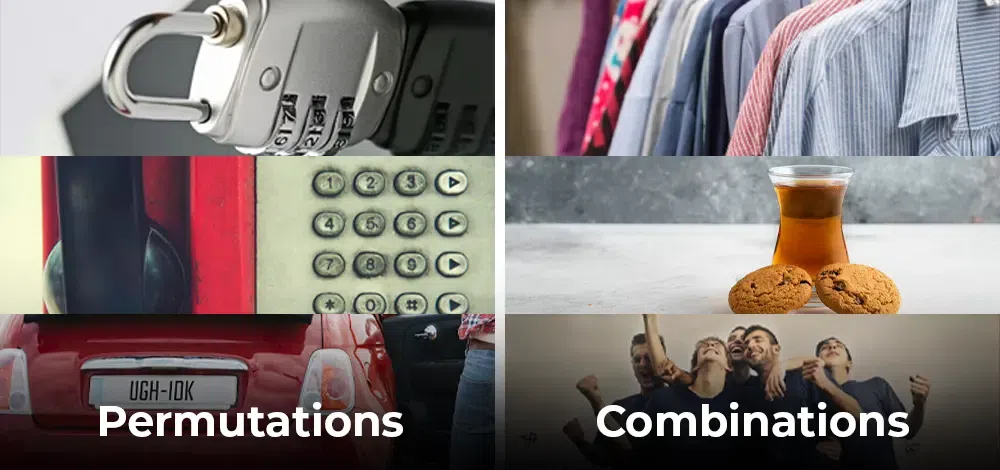## Permutation

Permutation is the distinct interpretations of a provided number of components carried one by one, or some, or all at a time. For example, if we have two components A and B, then there are two likely performances, AB and BA.

A numeral of permutations when ‘r’ components are positioned out of a total of ‘n’ components is n Pr. For example, let n = 3 (A, B, and C) and r = 2 (All permutations of size 2). Then there are 3P2 such permutations, which is equal to 6. These six permutations are AB, AC, BA, BC, CA, and CB. The six permutations of A, B, and C taken three at a time are shown in the image added below: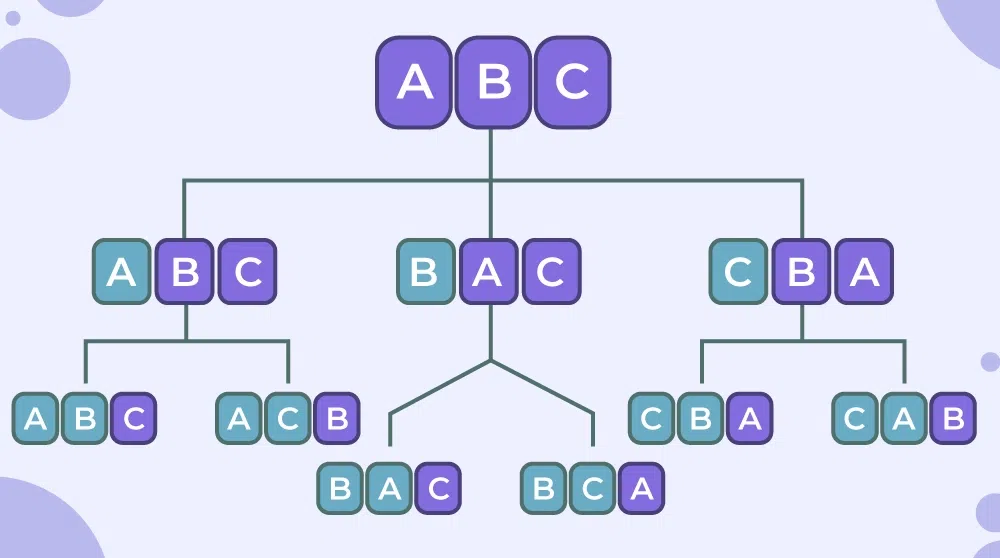### Permutation Formula

Permutation formula is used to find the number of ways to pick r things out of n different things in a specific order and replacement is not allowed and is given as follows: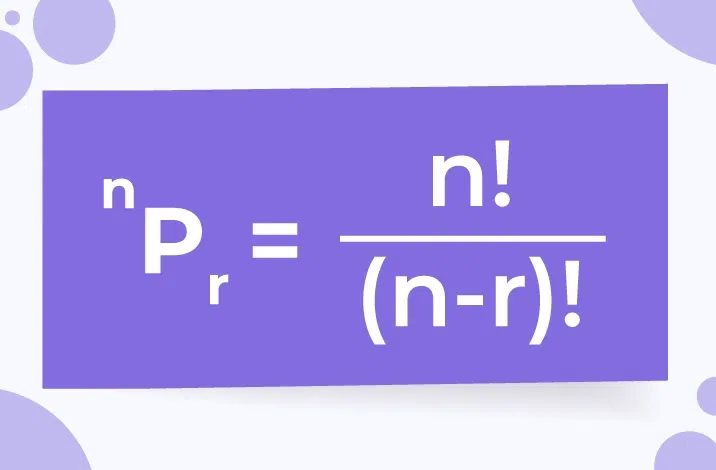### Explanation of Permutation Formula

As we know, permutation is a arrengement of r things out of n where order of arrengement is important( AB and BA are two different permutation). If there are three different numerals 1, 2 and 3 and if someone is curious to permute the numerals taking 2 at a moment, it shows (1, 2), (1, 3), (2, 1), (2, 3), (3, 1), and (3, 2). That is it can be accomplished in 6 methods.

Here, (1, 2) and (2, 1) are distinct. Again, if these 3 numerals shall be put handling all at a time, then the interpretations will be (1, 2, 3), (1, 3, 2), (2, 1, 3), (2, 3, 1), (3, 1, 2) and (3, 2, 1) i.e. in 6 ways.

In general, n distinct things can be set taking r (r < n) at a time in n(n – 1)(n – 2)…(n – r + 1) ways. In fact, the first thing can be any of the n things. Now, after choosing the first thing, the second thing will be any of the remaining n – 1 things. Likewise, the third thing can be any of the remaining n – 2 things. Alike, the rth thing can be any of the remaining n – (r – 1) things.

Hence, the entire number of permutations of n distinct things carrying r at a time is n(n – 1)(n – 2)…[n – (r – 1)] which is written as n Pr. Or, in other words,## Combination

It is the distinct sections of a shared number of components carried one by one, or some, or all at a time. For example, if there are two components A and B, then there is only one way to select two things, select both of them.

For example, let n = 3 (A, B, and C) and r = 2 (All combinations of size 2). Then there are 3C2 such combinations, which is equal to 3. These three combinations are AB, AC, and BC.

Here, the combination of any two letters out of three letters A, B, and C is shown below, we notice that in combination the order in which A and B are taken is not important as AB and BA represent the same combination.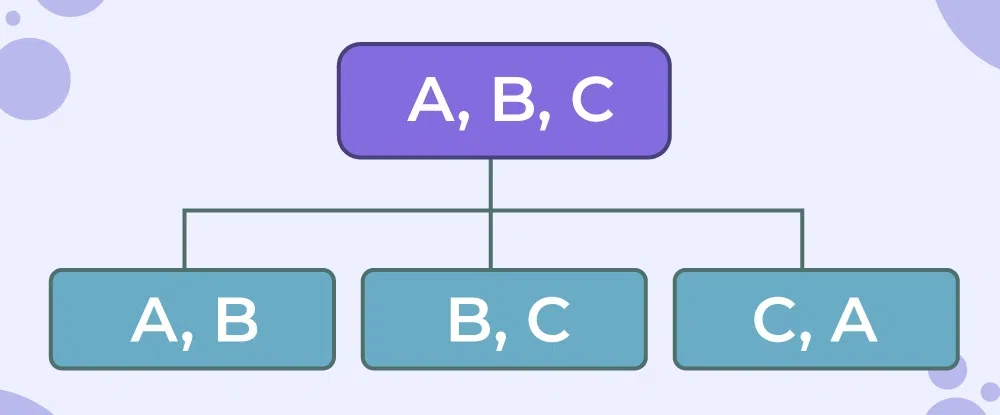Note: In the same example, we have distinct points for permutation and combination. For, AB and BA are two distinct items i.e., two distinct permutation, but for selecting, AB and BA are the same i.e., same combination.

### Combination Formula

Combination Formula is used to choose ‘r’ components out of a total number of ‘n’ components, and is given by: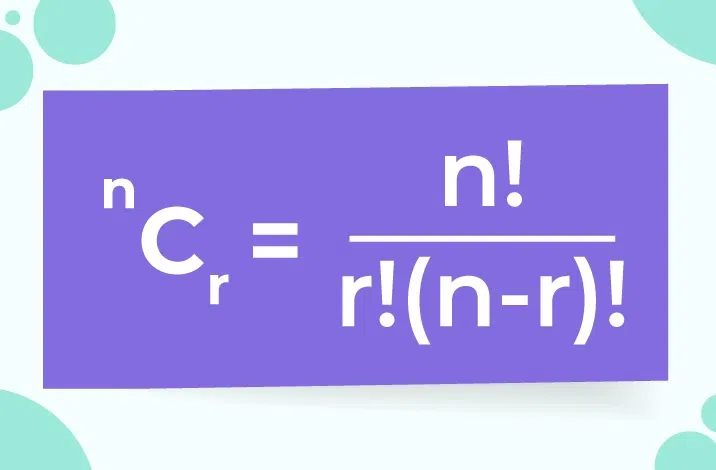Using the above formula for r and (n-r), we get the same result. Thus,### Explanation of Combination Formula

Combination, on the further hand, is a type of pack. Again, out of those three numbers 1, 2, and 3 if sets are created with two numbers, then the combinations are (1, 2), (1, 3), and (2, 3).

Here, (1, 2) and (2, 1) are identical, unlike permutations where they are distinct. This is written as 3C2. In general, the number of combinations of n distinct things taken r at a time is,## Derivation of Permutation and Combination Formulas

We can derive these Permutation and Combination formulas using the basic counting methods as these formulas represent the same thing. Derivation of these formulas is as follows:

### Derivation of Permutations Formula

Permutation is selecting r distinct objects from n objects without replacement and where the order of selection is important, by the fundamental theorem of counting and the definition of permutation, we get

P (n, r) = n . (n-1) . (n-2) . (n-3).  . . .  .(n-(r+1))

By Multiplying and Dividing above with (n-r)! = (n-r).(n-r-1).(n-r-2).  . . .  .3. 2. 1, we get

P (n, r) = [n.(n−1).(n−2)….(nr+1)[(n−r)(n−r−1)(n-r)!] / (n-r)!

⇒ P (n, r) = n!/(n−r)!

Thus, the formula for P (n, r) is derived.

### Derivation of Combinations Formula

Combination is choosing r items out of n items when the order of selection is of no importance. Its formula is calculated as,

C(n, r) = Total Number of Permutations /Number of ways to arrange r different objects.
[Since by the fundamental theorem of counting, we know that number of ways to arrange r different objects in r ways = r!]

C(n,r) = P (n, r)/ r!

⇒ C(n,r) = n!/(n−r)!r!

Thus, the formula for Combination i.e., C(n, r) is derived.

## Difference Between Permutation and Combination

Various differences between the permutation and combination can be understood by the following table:

## Solved Examples on Permutation and Combination

Example 1: Find the number of permutations and combinations of n = 9 and r = 3.

Solution:

Given, n = 9, r = 3

Using the formula given above:

For Permutation:

nPr = (n!) / (n – r)!

nPr = (9!) / (9 – 3)!

nPr = 9! / 6! = (9 × 8 × 7 × 6! )/ 6!

nPr = 504

For Combination:

nCr = n!/r!(n − r)!

nCr = 9!/3!(9 − 3)!

nCr = 9!/3!(6)!

nCr = 9 × 8 × 7 × 6!/3!(6)!

nCr = 84

Example 2: In how many ways a committee consisting of 4 men and 2 women, can be chosen from 6 men and 5 women?

Solution:

Choose 4 men out of 6 men = 6C4 ways = 15 ways

Choose 2 women out of 5 women = 5C2 ways = 10 ways

The committee can be chosen in 6C4 × 5C2  = 150 ways.

Example 3: In how many ways can 5 different books be arranged on a shelf?

Solution:

This is a permutation problem because the order of the books matters.

Using the permutation formula, we get:

5P5 = 5! / (5 – 5)! = 5! / 0! = 5 x 4 x 3 x 2 x 1 = 120

Therefore, there are 120 ways to arrange 5 different books on a shelf.

Example 4: How many 3-letter words can be formed using the letters from the word “FABLE”?

Solution:

This is a permutation problem because the order of the letters matters.

Using the permutation formula, we get:

5P3 = 5! / (5 – 3)! = 5! / 2! = 5 x 4 x 3 = 60

Therefore, there are 60 3-letter words that can be formed using the letters from the word “FABLE”.

Example 5: A committee of 5 members is to be formed from a group of 10 people. In how many ways can this be done?

Solution:

This is a combination problem because the order of the members doesn’t matter.

Using the combination formula, we get:

10C5 = 10! / (5! x (10 – 5)!) = 10! / (5! x 5!)

10C5= (10 x 9 x 8 x 7 x 6) / (5 x 4 x 3 x 2 x 1) = 252

Therefore, there are 252 ways to form a committee of 5 members from a group of 10 people.

Example 6: A pizza restaurant offers 4 different toppings for their pizzas. If a customer wants to order a pizza with exactly 2 toppings, in how many ways can this be done?

Solution:

This is a combination problem because the order of the toppings doesn’t matter.

Using the combination formula, we get:

4C2 = 4! / (2! x (4 – 2)!) = 4! / (2! x 2!) = (4 x 3) / (2 x 1) = 6

Therefore, there are 6 ways to order a pizza with exactly 2 toppings from 4 different toppings.

Example 7: How considerable words can be created by using 2 letters from the term“LOVE”?

Solution:

The term “LOVE” has 4 distinct letters.

Therefore, required number of words = 4P2 = 4! / (4 – 2)!

Required number of words = 4! / 2! = 24 / 2

⇒ Required number of words = 12

Example 8: Out of 5 consonants and 3 vowels, how many words of 3 consonants and 2 vowels can be formed?

Solution:

Number of ways of choosing 3 consonants from 5 = 5C3

Number of ways of choosing 2 vowels from 3 = 3C2

Number of ways of choosing 3 consonants from 2 and 2 vowels from 3 = 5C3 × 3C2

⇒ Required number = 10 × 3

= 30

It means we can have 30 groups where each group contains a total of 5 letters (3 consonants and 2 vowels).

Number of ways of arranging 5 letters among themselves

= 5! = 5 × 4 × 3 × 2 × 1 = 120

Hence, the required number of ways = 30 × 120

⇒ Required number of ways = 3600

Example 9: How many different combinations do you get if you have 5 items and choose 4?

Solution:

Insert the given numbers into the combinations equation and solve. “n” is the number of items that are in the set (5 in this example); “r” is the number of items you’re choosing (4 in this example):

C(n, r) = n! / r! (n – r)!

nCr = 5! / 4! (5 – 4)!

nCr = (5 × 4 × 3 × 2 × 1) / (4 × 3 × 2 × 1 × 1)

nCr = 120/24

nCr = 5

The solution is 5.

Example 10: Out of 6 consonants and 3 vowels, how many expressions of 2 consonants and 1 vowel can be created?

Solution:

Number of ways of selecting 2 consonants from 6 = 6C2

Number of ways of selecting 1 vowels from 3 = 3C1

Number of ways of selecting 3 consonants from 7 and 2 vowels from 4.

⇒ Required ways = 6C2 × 3C1

⇒ Required ways = 15 × 3

⇒ Required ways= 45

It means we can have 45 groups where each group contains a total of 3 letters (2 consonants and 1 vowels).

Number of ways of arranging 3 letters among themselves = 3! = 3 × 2 × 1

⇒ Required ways to arrenge three letters = 6

Hence, the required number of ways = 45 × 6

⇒ Required ways = 270

Example 11: In how many distinct forms can the letters of the term ‘PHONE’ be organized so that the vowels consistently come jointly?

Solution:

The word ‘PHONE’ has 5 letters. It has the vowels ‘O’,’ E’, in it and these 2 vowels should consistently come jointly. Thus these two vowels can be grouped and viewed as a single letter. That is, PHN(OE).

Therefore we can take total letters like 4 and all these letters are distinct.

Number of methods to organize these letters = 4! = 4 × 3 × 2 × 1

⇒ Required ways arrenge letters = 24

All the 2 vowels (OE) are distinct.

Number of ways to arrange these vowels among themselves = 2! = 2 × 1

⇒ Required ways to arrange vowels = 2

Hence, the required number of ways = 24 × 2

⇒ Required ways = 48.

## FAQs on Permutations and Combinations

### Q1: What is the factorial formula?

Factorial formula is used for the calculation of permutations and combinations. The factorial formula for n! is given as

n! = n × (n-1) × . . . × 4 × 3 × 2 × 1

For example, 3! = 3 × 2 × 1 = 6 and 5! = 5 × 4 × 3 × 2 × 1 = 120.

### Q2: What does nCr represent?

nCr represents the number of combinations that can be made from “n” objects taking “r” at a time.

### Q3: What do you mean by permutations and combinations?

A permutation is an act of arranging things in a specific order. Combinations are the ways of selecting r objects from a group of n objects, where the order of the object chosen does not affect the total combination.

### Q4: Write examples of permutations and combinations.

The number of 3-letter words that can be formed by using the letters of the word says, HELLO; 5P3 = 5!/(5-3)! this is an example of a permutation.
The number of combinations we can write the words using the vowels of the word HELLO; 5C2 =5!/[2! (5-2)!], this is an example of a combination.

### Q5: Write the formula for finding permutations and combinations.

• Formula for calculating permutations: nPr = n!/(n-r)!
• Formula for calculating combinations: nCr = n!/[r! (n-r)!]